# Arithmetic series

(diff) ← Older revision | Latest revision (diff) | Newer revision → (diff)

of orderThe sequence of values of a polynomial of degree: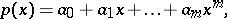assumed by the polynomial when the variabletakes successive integral non-negative values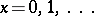. If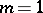, i.e.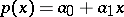, one obtains an arithmetic progression with initial term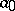and difference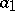. If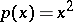or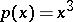, one obtains sequences of squares or cubes of integers, i.e. special cases of arithmetic series of the second and third orders. If a first difference series is created, constituted by the differences between successive terms of an arithmetic series, then a series of differences of the first difference series (second differences) is written, and then the second differences are used to form third differences, etc., one finds, at the-th stage, that all-th differences are equal. Conversely, if the-th differences of a numerical sequence are all equal, the sequence is an arithmetic series of order. Using this property, it is possible to construct arithmetic series of different orders from their differences. For example, the sequence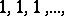may be regarded as the first differences of the series of natural numbers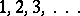; as the second differences of the series of triangular numbers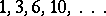; as the third differences of the sequence of tetrahedral numbers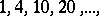etc. These numbers are so called because triangular numbers represent numbers arranged in the form of a triangle (Fig. a), while tetrahedral numbers represent numbers arranged in the form of tetrahedra (pyramids) (Fig. b).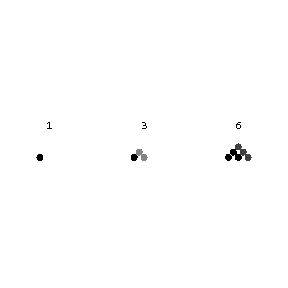Figure: a013370a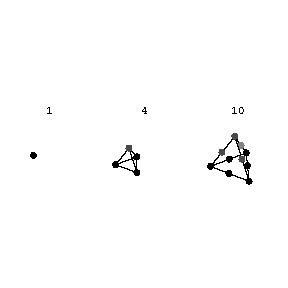Figure: a013370b

Triangular numbers are expressed by the formula, while tetrahedral numbers are given by the formula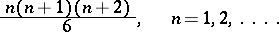A generalization of triangular numbers is constituted by-gonal or figurate numbers, which played an important role in the development of arithmetic in its various stages.Figure: a013370c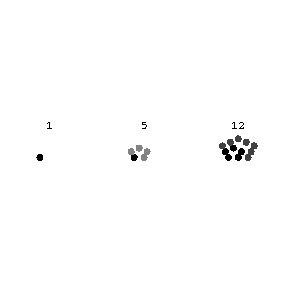Figure: a013370d-gonal numbers are given by the formula: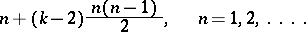They form an arithmetic series of the second order, with one as their first term,as their second term and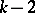as their second differences. If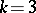, triangular numbers are obtained; if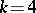, one obtains squares (); if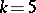, one obtains pentagonal numbers, etc. These appellations will become clear from Fig. cand Fig. d, in which the number of beads arranged in the form of a square or a pentagon is expressed by the respective square or pentagonal number. Figurate numbers satisfy the following theorem, proposed by P. Fermat and first proved by A.L. Cauchy: Any natural number can be represented as a sum of not more than-gonal numbers.

How to Cite This Entry:
Arithmetic series. Encyclopedia of Mathematics. URL: http://encyclopediaofmath.org/index.php?title=Arithmetic_series&oldid=15146
This article was adapted from an original article by BSE-2 (originator), which appeared in Encyclopedia of Mathematics - ISBN 1402006098. See original article